## General Rules for Assigning Electrons to Atomic Orbitals

An atom’s electrons exist in discrete atomic orbitals, and the atom’s electron configuration can be determined using a set of guidelines.

### Learning Objectives

Determine the electron configuration for elements and ions, identifying the relation between electron shells and subshells.

### Key Takeaways

#### Key Points

• If the energy of an atom is increased, an electron in the atom gets excited. To go back to its ground state, the electron releases energy. The energy of the light released when an electron drops in energy level is the same as the difference in energy between the two levels.
• Viewed simply, electrons are arranged in shells around an atom’s nucleus. Electrons closest to the nucleus will have the lowest energy. Electrons further away from the nucleus will have higher energy. An atom’s electron shell can accommodate 2n2 electrons (where n is the shell level).
• In a more realistic model, electrons move in atomic orbitals, or subshells. There are four different orbital shapes: s, p, d, and f. Within each shell, the s subshell is at a lower energy than the p. An orbital diagram is used to determine an atom’s electron configuration.
• There are guidelines for determining the electron configuration of an atom. An electron will move to the orbital with lowest energy. Each orbital can hold only one electron pair. Electrons will separate as much as possible within a shell.

#### Key Terms

• frequency: The number of occurrences of a repeating event per unit of time.
• quantization: The process of approximating a continuous signal by a set of discrete symbols or integer values.

### Energy of Electrons in Atomic Orbitals

The central structure of an atom is the nucleus, which contains protons and neutrons. This nucleus is surrounded by electrons. Although these electrons all have the same charge and the same mass, each electron in an atom has a different amount of energy. Electrons with the lowest energy are found closest to the nucleus, where the attractive force of the positively charged nucleus is the greatest. Electrons that have higher energy are found further away.

### Energy Quantization

When the energy of an atom is increased (for example, when a substance is heated), the energy of the electrons inside the atom is also increased—that is to say, the electrons get excited. For the excited electron to go back to its original energy, or ground state, it needs to release energy. One way an electron can release energy is by emitting light. Each element emits light at a specific frequency (or color) upon heating that corresponds to the energy of the electronic excitation.

It is helpful to think of this like going up a flight of steps. If you don’t lift your foot enough, you will bump into the step and be stuck on the ground level. You need to lift your foot to the height of the step to move on. The same goes for electrons and the amount of energy they can have. This separating of electrons into energy units is called quantization of energy because there are only certain quantities of energy that an electron can have in an atom. The energy of the light released when an electron drops down from a higher energy level to a lower energy level is the same as the difference in energy between the two levels.

### Electron Shells

We will start with a very simple way of showing the arrangement of electrons around an atom. Here, electrons are arranged in energy levels, or shells, around the nucleus of an atom. Electrons that are in the first energy level (energy level 1) are closest to the nucleus and will have the lowest energy. Electrons further away from the nucleus will have higher energy. An atom’s electron shell can accommodate 2n2 electrons, where n is the energy level. For example, the first shell can accommodate 2 x (1)2 or 2 electrons. The second shell can accommodate 2 x (2)2, or 8, electrons.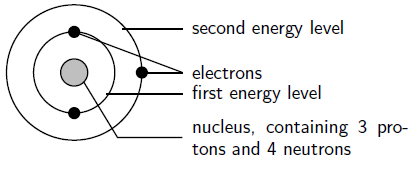The arrangement of electrons in a lithium atom: Lithium (Li) has an atomic number of 3, meaning that in a neutral atom, the number of electrons will be 3. The energy levels are shown as concentric circles around the central nucleus, and the electrons are placed from the inside out. The first two electrons are found in the first energy level, and the third electron is found in the second energy level.

As an example, fluorine (F), has an atomic number of 9, meaning that a neutral fluorine atom has 9 electrons. The first 2 electrons are found in the first energy level, and the other 7 are found in the second energy level.

### Atomic Orbitals

Though electrons can be represented simply as circling the nucleus in rings, in reality, electrons move along paths that are much more complicated. These paths are called atomic orbitals, or subshells. There are several different orbital shapes—s, p, d, and f—but we will be focusing mainly on s and p orbitals for now. The first energy level contains only one s orbital, the second energy level contains one s orbital and three p orbitals, and the third energy level contains one s orbital, three p orbitals, and five d orbitals. Within each energy level, the s orbital is at a lower energy than the p orbitals.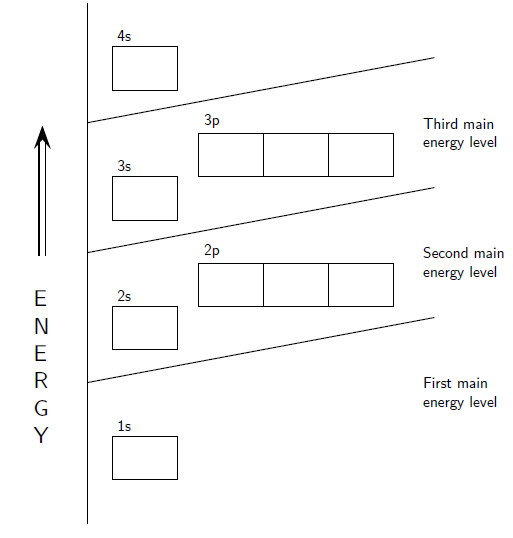Orbital diagram: The positions of the first ten orbits of an atom on an energy diagram. Note that each block is able to hold two electrons.

An orbital diagram helps to determine the electron configuration of an element. An element’s electron configuration is the arrangement of the electrons in the shells. There are a few guidelines for working out this configuration:

• Each orbital can hold only two electrons. Electrons that occur together in an orbital are called an electron pair.
• An electron will always try to enter the orbital with the lowest energy.
• An electron can occupy an orbital on its own, but it would rather occupy a lower-energy orbital with another electron before occupying a higher-energy orbital. In other words, within one energy level, electrons will fill an s orbital before starting to fill p orbitals.
• The s subshell can hold 2 electrons.
• The p subshells can hold 6 electrons.

Electron configurations can be used to rationalize chemical properties in both inorganic and organic chemistry. It is also used to interpret atomic spectra, the method used to measure the energy of light emitted from elements and compounds.

## The Building-Up (Aufbau) Principle

The Aufbau principle determines an atom’s electron configuration by adding electrons to atomic orbitals following a defined set of rules.

### Learning Objectives

Write electron configurations for elements in standard notation.

### Key Takeaways

#### Key Points

• The Madelung rule defines the order in which atomic orbitals are filled with electrons. Electrons fill orbitals starting at the lowest available energy state before filling higher states.
• Aufbau procedure: Determine number of electrons for the atom of interest. Fill available orbitals starting with the lowest-energy levels first and avoid pairing electrons in a single orbital until it is necessary.
• Electron configuration notation describes the energy levels, orbitals, and the number of electron. The number and letter describe the energy level and orbital respectively, and the superscript number shows how many electrons are in that orbital.
• The Aufbau principle works well for the first 18 elements but then becomes less useful.

#### Key Terms

• Pauli Exclusion Principle: The quantum mechanical principle that no two identical fermions (particles with half-integer spin) may occupy the same quantum state simultaneously.

### Atoms Are Built Up by Adding Electrons

Although the nucleus of an atom is very dense, the electrons around it can take on a variety of positions which can be summarized as an electron configuration. An element’s electron configuration can be represented using energy level diagrams, or Aufbau diagrams. The Aufbau principle (from the German Aufbau meaning “building up, construction”) describes a model-building method in which an atom is “built up” by progressively adding electrons. As electrons are added, they assume the most stable shells with respect to the nucleus and the electrons already present.

### Filling in an Aufbau Diagram

The order in which orbitals are filled is given by the Madelung rule. The rule is based on the total number of nodes in the atomic orbital, n + ℓ, which is related to the energy. In this context, n represents the principal quantum number and ℓ represents the azimuthal quantum number. The values ℓ = 0, 1, 2, 3 correspond to the s, p, d, and f labels, respectively. According to the principle, electrons fill orbitals starting at the lowest available energy states before filling higher states (e.g., 1s before 2s).The Madelung energy ordering rule: Order in which orbitals are arranged by increasing energy according to the Madelung Rule. Each diagonal read arrow corresponds to a different value of n + l.

An Aufbau diagram uses arrows to represent electrons. When there are two electrons in an orbital, the electrons are called an electron pair. Electron pairs are shown with arrows pointing in opposite directions. According to the Pauli Exclusion Principle, two electrons in an orbital will not spin the same way. That is, an Aufbau diagram uses arrows pointing in opposite directions. An arrow pointing up denotes an electron spinning one way and an arrow pointing downwards denotes an electron spinning the other way. If the orbital only has one electron, this electron is called an unpaired electron.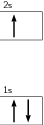Aufbau diagram for lithium: The electron configuration of lithium, shown on an Aufbau diagram

The following steps detail how to draw an Aufbau diagram:

1. Determine the number of electrons that the atom has.
2. Fill the s orbital in the first energy level (the 1s orbital) with the first two electrons.
3. Fill the s orbital in the second energy level (the 2s orbital) with the second two electrons.
4. Put one electron in each of the three p orbitals in the second energy level (the 2p orbitals) and then if there are still electrons remaining, go back and place a second electron in each of the 2p orbitals to complete the electron pairs.
5. Continue in this way through each of the successive energy levels until all the electrons have been drawn.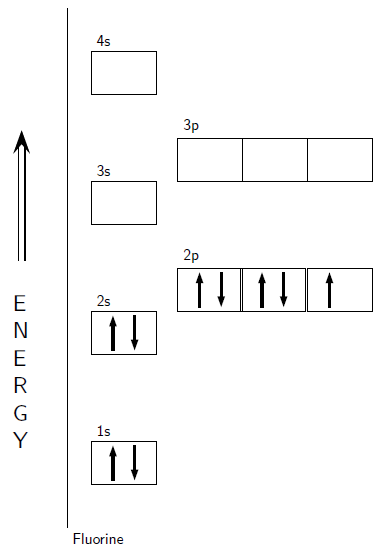Aufbau diagram for fluorine: An Aufbau diagram showing the electron configuration of fluorine.

### Electron Configuration Standard Notation

A special type of notation is used to write an atom’s electron configuration. The notation describes the energy levels, orbitals, and the number of electrons in each. For example, the electron configuration of lithium is 1s22s1. The number and letter describe the energy level and orbital, and the number above the orbital shows how many electrons are in that orbital. Using standard notation, the electron configuration of fluorine is 1s22s22p5.

### Limitations to Aufbau

The Aufbau principle is based on the idea that the order of orbital energies is fixed—both for a given element and between different elements. This assumption is approximately true—enough for the principle to be useful—but not physically reasonable. It models atomic orbitals as “boxes” of fixed energy into which at most two electrons can be placed. However, the energy of an electron in an atomic orbital depends on the energies of all the other electrons of the atom.

In a hydrogen-like atom, which only has one electron, the s-orbital and the p-orbitals of the same shell in the Aufbau diagram have exactly the same energy. However, in a real hydrogen atom, the energy levels are slightly split by the magnetic field of the nucleus. Because each atom has a different number of protons in its nucleus, the magnetic field differs, which alters the pull on each electron. In general, the Aufbau principle works very well for the ground states of the atoms for the first 18 elements, then decreasingly well for the following 100 elements.

Interactive: Energy Levels of a Hydrogen Atom: The likely location of an electron around the nucleus of an atom is called an orbital. The shape of an orbital depends on the energy state of the electron. A neutral hydrogen atom has one electron. Click in the boxes to set the energy of that electron and see the orbital shape describing where you are likely to find that electron around the nucleus.

## Hund’s Rule

Hund’s Rule defines the behavior of unpaired valence shell electrons, providing insight into an atom’s reactivity and stability.

### Learning Objectives

Apply Hund’s rule and justify its use to determine electron configurations for atoms in the ground state

### Key Takeaways

#### Key Points

• Hund’s Rule states that every orbital in a sublevel is singly occupied before any orbital is doubly occupied and all of the electrons in singly occupied orbitals have the same spin.
• Electrons arrange themselves in order to minimize their interaction energy. They will always occupy an empty orbital before they pair up to minimize repulsion. Unpaired electrons have the same spins because they meet less often if traveling in the same direction than if traveling in opposite directions.
• To avoid confusion, scientists always draw the first electron, and any other unpaired electron, in an orbital as “spin-up.”
• Electron configurations help make predictions about how certain elements will react. An atom is most reactive when its valence shell is not full and most stable when its valence orbitals are full. Elements that have the same number of valence electrons often have similar properties.

#### Key Terms

• repulsion: A force that moves two bodies away from each other.
• hund’s rule: A rule which states that a greater total spin state usually makes the resulting atom more stable.
• quantum-mechanical calculation: Branch of physics which studies matter and energy at the level of atoms and other elementary particles, and substitutes probabilistic mechanisms for classical Newtonian ones.

### Relation to Aufbau Principle

Electrons will fill the lowest energy orbitals first and then move up to higher energy orbitals only after the lower energy orbitals are full. This is referred to as the Aufbau Principle, after the scientist who proposed the concept. Although the implications are clear for orbitals of different principal quantum number (n), which are clearly of different energy, the filling order is less clear for degenerate sublevels. For example, for boron through neon, the electron filling order of the 2p orbitals follows Hund’s Rule.

Hund’s Rule states that:

1. Every orbital in a sublevel is singly occupied before any orbital is doubly occupied.
2. All of the electrons in singly occupied orbitals have the same spin.

### Hund’s Rule Explained

According to the first rule, electrons will always occupy an empty orbital before they pair up. Electrons are negatively charged and, as a result, they repel each other. Electrons tend to minimize repulsion by occupying their own orbital, rather than sharing an orbital with another electron. Further, quantum-mechanical calculations have shown that the electrons in singly occupied orbitals are less effectively screened or shielded from the nucleus.

For the second rule, unpaired electrons in singly occupied orbitals have the same spins. If all electrons are orbiting in the same direction, they meet less often than if some of them orbit in opposite directions. In the latter case, the repulsive force increases, which separates electrons. Therefore, spins that are aligned have lower energy.

Technically speaking, the first electron in a sublevel could be either “spin-up” or “spin-down.” Once the spin of the first electron in a sublevel is chosen, the spins of all of the other electrons in that sublevel depend on that first choice. To avoid confusion, scientists always draw the first electron, and any other unpaired electron, in an orbital as “spin-up.”

### Applying Hund’s Rule

For example, take the electron configuration for carbon: 2 electrons will pair up in the 1s orbital, 2 electrons pair up in the 2s orbital, and the remaining 2 electrons will be placed into the 2p orbitals. The correct orbital diagram, obeying Hund’s Rule, will note the two 2p electrons to be unpaired in two of the three available orbitals, both with “spin-up.” Since electrons always occupy an empty orbital before they fill up, it would be incorrect to draw the two 2p electrons in the same orbital, leaving open orbitals unfilled.Example of Hund’s rule: Orbital diagram for carbon, showing the correct application of Hund’s Rule.

As another example, oxygen has 8 electrons. The electron configuration can be written as 1s22s22p4. The orbital diagram is drawn as follows: the first 2 electrons will pair up in the 1s orbital; the next 2 electrons will pair up in the 2s orbital. That leaves 4 electrons, which must be placed in the 2p orbitals. According to Hund’s Rule, all orbitals will be singly occupied before any is doubly occupied. Therefore, two p orbitals will each get 1 electron and one will get 2 electrons. Hund’s Rule also tells us that all of the unpaired electrons must have the same spin. Keeping with convention, all of the unpaired electrons are drawn as “spin-up.”Application of Hund’s rule: Orbital diagram for oxygen, which has four 2p electrons, showing the correct application of Hund’s Rule.

### Purpose of Electron Configurations

When atoms come into contact with one another, it is the outermost electrons of these atoms, or valence shell, that will interact first. An atom is least stable (and therefore most reactive) when its valence shell is not full. The valence electrons are largely responsible for an element’s chemical behavior. Elements that have the same number of valence electrons often have similar chemical properties.

Electron configurations can also predict stability. An atom is at its most stable (and therefore unreactive) when all its orbitals are full. The most stable configurations are the ones that have full energy levels. These configurations occur in the noble gases. The noble gases are very stable elements that do not react easily with any other elements.

Electron configurations can help to make predictions about the ways in which certain elements will react and the chemical compounds or molecules that different elements will form. These principles help to understand the behavior of all chemicals, from the most basic elements like hydrogen and helium, to the most complex proteins (huge biological chemicals made of thousands of different atoms bound together) found in the human body.

## The Shielding Effect and Effective Nuclear Charge

The shielding effect, approximated by the effective nuclear charge, is due to inner electrons shielding valence electrons from the nucleus.

### Learning Objectives

Calculate effective nuclear charges experienced by valence electrons.

### Key Takeaways

#### Key Points

• The shielding effect describes the balance between the pull of the protons on valence electrons and the repulsion forces from inner electrons.
• The shielding effect explains why valence-shell electrons are more easily removed from the atom. The effect also explains atomic size. The more shielding, the further the valence shell can spread out and the bigger atoms will be.
• The effective nuclear charge is the net positive charge experienced by valence electrons. It can be approximated by the equation: Zeff = Z – S, where Z is the atomic number and S is the number of shielding electrons.

#### Key Terms

• cation: A positively charged ion, as opposed to an anion.
• valence shell: The outermost shell of electrons in an atom; these electrons take part in bonding with other atoms.
• valence shell electron pair repulsion theory: A set of rules used to predict the shape of individual molecules.
• core electrons: Those that are not part of the valence shell and as such, are not involved in bonding.
• nucleus: The positively charged central part of an atom, made up of protons and neutrons.
• effective nuclear charge: That experienced by an electron in a multi-electron atom, typically less for electrons that are shielded by core electrons.
• anion: A negatively charged ion, as opposed to a cation.

### The Shielding Effect

Electrons in an atom can shield each other from the pull of the nucleus. This effect, called the shielding effect, describes the decrease in attraction between an electron and the nucleus in any atom with more than one electron shell. The more electron shells there are, the greater the shielding effect experienced by the outermost electrons.

In hydrogen-like atoms, which have just one electron, the net force on the electron is as large as the electric attraction from the nucleus. However, when more electrons are involved, each electron (in the n-shell) feels not only the electromagnetic attraction from the positive nucleus but also repulsion forces from other electrons in shells from 1 to n-1. This causes the net electrostatic force on electrons in outer shells to be significantly smaller in magnitude. Therefore, these electrons are not as strongly bound as electrons closer to the nucleus.

The shielding effect explains why valence shell electrons are more easily removed from the atom. The nucleus can pull the valence shell in tighter when the attraction is strong and less tight when the attraction is weakened. The more shielding that occurs, the further the valence shell can spread out. As a result, atoms will be larger.

### Example

Why is cesium bigger than elemental sodium?

The element sodium has the electron configuration 1s22s22p63s1. The outer energy level is n = 3 and there is one valence electron. The attraction between this lone valence electron and the nucleus with 11 protons is shielded by the other 10 core electrons.

The electron configuration for cesium is 1s22s22p63s23p64s23d104p65s24d105p66s1. While there are more protons in a cesium atom, there are also many more electrons shielding the outer electron from the nucleus. The outermost electron, 6s1, therefore, is held very loosely. Because of shielding, the nucleus has less control over this 6s1 electron than it does over a 3s1 electron.

### Effective Nuclear Charge

The magnitude of the shielding effect is difficult to calculate precisely. As an approximation, we can estimate the effective nuclear charge on each electron.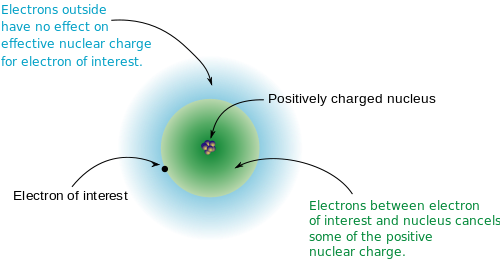Effective nuclear charge diagram: Diagram of the concept of effective nuclear charge based on electron shielding.

The effective nuclear charge (often symbolized as Zeff or Z*) is the net positive charge experienced by an electron in a multi-electron atom. The term “effective” is used because the shielding effect of negatively charged electrons prevents higher orbital electrons from experiencing the full nuclear charge.

The effective nuclear charge on an electron is given by the following equation:

Zeff = Z – S

where Z is the number of protons in the nucleus (atomic number), and S is the number of electrons between the nucleus and the electron in question (the number of nonvalence electrons).

### Example

Consider a neutral neon atom (Ne), a sodium cation (Na+), and a fluorine anion (F). What is the effective nuclear charge for each?

Start by figuring out the number of nonvalence electrons, which can be determined from the electron configuration.

Ne has 10 electrons. The electron configuration is 1s22s2 2p6. The valence shell is shell 2 and contains 8 valence electrons. Thus the number of nonvalence electrons is 2 (10 total electrons – 8 valence). The atomic number for neon is 10, therefore:

Zeff(Ne) = 10 – 2 = 8+

Flourine has 9 electrons but F has gained an electron and thus has 10. The electron configuration is the same as for neon and the number of nonvalence electrons is 2. The atomic number for F is 9, therefore:

Zeff(F) = 9 – 2 = 7+

Sodium has 11 electrons but the Na+ ion has lost an electron and thus has 10. Once again, the electron configuration is the same as in the previous examples and the number of nonvalence electrons is 2 (by losing one electron, the valence shell becomes the n=2 shell). The atomic number for Na+ is 11, therefore:

Zeff(Na+) = 11 – 2 = 9+

In each of the above examples (Ne, F, Na+) an atom has 10 electrons but the effective nuclear charge varies because each has a different atomic number. The sodium cation has the largest effective nuclear charge, which results in electrons being held the tightest, and therefore Na+ has the smallest atomic radius.

## Diamagnetism and Paramagnetism

Diamagnetic atoms have only paired electrons, whereas paramagnetic atoms, which can be made magnetic, have at least one unpaired electron.

### Learning Objectives

Distinguish diamagnetic from paramagnetic atoms.

### Key Takeaways

#### Key Points

• Any time two electrons share the same orbital, their spin quantum numbers have to be different. Whenever two electrons are paired together in an orbital, or their total spin is 0, they are diamagnetic electrons. Atoms with all diamagnetic electrons are called diamagnetic atoms.
• A paramagnetic electron is an unpaired electron. An atom is considered paramagnetic if even one orbital has a net spin. An atom could have ten diamagnetic electrons, but as long as it also has one paramagnetic electron, it is still considered a paramagnetic atom.
• Diamagnetic atoms repel magnetic fields. The unpaired electrons of paramagnetic atoms realign in response to external magnetic fields and are therefore attracted. Paramagnets do not retain magnetization in the absence of a magnetic field, because thermal energy randomizes electron spin orientations.

#### Key Terms

• quantum number: One of certain integers or half-integers that specify the state of a quantum mechanical system (such as an electron in an atom).
• lanthanide: Any of the 14 rare earth elements from cerium (or from lanthanum) to lutetium in the periodic table. Because their outermost orbitals are empty, they have very similar chemistry. Below them are the actinides.
• diamagnetic: Materials that create an induced magnetic field in a direction opposite to an externally applied magnetic field and are therefore repelled by the applied magnetic field.
• paramagnetic: Materials that are attracted by an externally applied magnetic field and form internal, induced magnetic fields in the direction of the applied magnetic field.
• MRI: Magnetic Resonance Imaging, a medical imaging technique used in radiology to investigate the anatomy and physiology of the body in both health and disease.

### Diamagnetism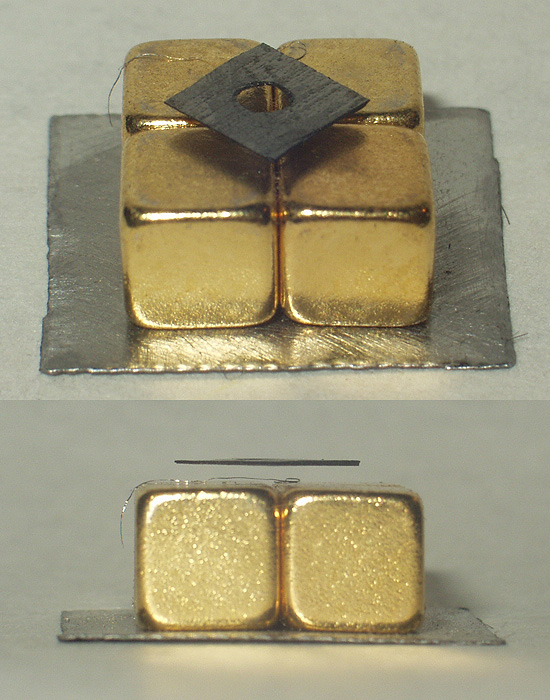Diamagnetic levitation: A small (~6mm) piece of pyrolytic graphite (a material similar to graphite) levitating over a permanent gold magnet array (5mm cubes on a piece of steel). Note that the poles of the magnets are aligned vertically and alternate (two with north facing up, and two with south facing up, diagonally).

Any time two electrons share the same orbital, their spin quantum numbers have to be different. In other words, one of the electrons has to be “spin-up,” with $m_s = +\frac{1}{2}$, while the other electron is “spin-down,” with $m_s = -\frac{1}{2}$. This is important when it comes to determining the total spin in an electron orbital. In order to decide whether electron spins cancel, add their spin quantum numbers together. Whenever two electrons are paired together in an orbital, or their total spin is 0, they are called diamagnetic electrons.

Think of spins as clockwise and counterclockwise. If one spin is clockwise and the other is counterclockwise, then the two spin directions balance each other out and there is no leftover rotation. Note what all of this means in terms of electrons sharing an orbital: Since electrons in the same orbital always have opposite values for their spin quantum numbers (ms), they will always end up canceling each other out. In other words, there is no leftover spin in an orbital that contains two electrons.

Electron spin is very important in determining the magnetic properties of an atom. If all of the electrons in an atom are paired up and share their orbital with another electron, then the total spin in each orbital is zero and the atom is diamagnetic. Diamagnetic atoms are not attracted to a magnetic field, but rather are slightly repelled.

### Paramagnetism

Electrons that are alone in an orbital are called paramagnetic electrons. Remember that if an electron is alone in an orbital, the orbital has a net spin, because the spin of the lone electron does not get canceled out. If even one orbital has a net spin, the entire atom will have a net spin. Therefore, an atom is considered to be paramagnetic when it contains at least one paramagnetic electron. In other words, an atom could have 10 paired (diamagnetic) electrons, but as long as it also has one unpaired (paramagnetic) electron, it is still considered a paramagnetic atom.

Just as diamagnetic atoms are slightly repelled from a magnetic field, paramagnetic atoms are slightly attracted to a magnetic field. Paramagnetic properties are due to the realignment of the electron paths caused by the external magnetic field. Paramagnets do not retain any magnetization in the absence of an externally applied magnetic field, because thermal motion randomizes the spin orientations. Stronger magnetic effects are typically only observed when d- or f-electrons are involved. The size of the magnetic moment on a lanthanide atom can be quite large, as it can carry up to seven unpaired electrons, in the case of gadolinium(III) (hence its use in MRI).